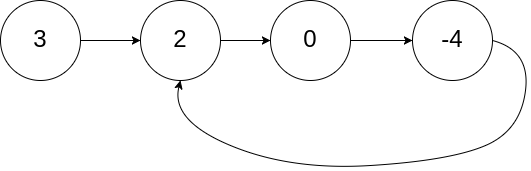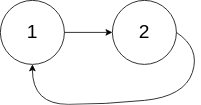# 142. Linked List Cycle II (Medium)

Given a linked list, return the node where the cycle begins. If there is no cycle, return `null`.

To represent a cycle in the given linked list, we use an integer `pos` which represents the position (0-indexed) in the linked list where tail connects to. If `pos` is `-1`, then there is no cycle in the linked list.

Note: Do not modify the linked list.

Example 1:

```Input: head = [3,2,0,-4], pos = 1
Output: tail connects to node index 1
Explanation: There is a cycle in the linked list, where tail connects to the second node.
```Example 2:

```Input: head = [1,2], pos = 0
Output: tail connects to node index 0
Explanation: There is a cycle in the linked list, where tail connects to the first node.
```Example 3:

```Input: head = , pos = -1
Output: no cycle
Explanation: There is no cycle in the linked list.
```Can you solve it without using extra space?

## Solutions

``````public class Solution {
// |----x1-----|--p-x2---|
// x1 represent the normal section of the list without circle, x2 represents the circle section of the list
// Assume step length of slow is 1, fast encounters slow after slow walks S steps.
// We can derive that the length slow walked is S, and fast is 2*S
// Suppose fast and slow meets at point p, subject to (2*S-x1)%x2 = (S-x1)%x2=p-x1
// A simplified formula of above is S%x2=0

// Then slow continues to proceed forward x1 steps, the finally position is x1+(S+x1-x1)%x2=?
// The simplified equation is x1+S%x2==x1+0=x1, that explains why after walking x1 steps(x1 length)
// slow2 meets slow at the start of the circle

while (fast != null && fast.next != null) {
slow = slow.next;

// even step size
fast = fast.next.next;

if (fast != slow) {
continue;
}

// fast == slow means both pointer fast and slow already run into a circle
// and the pointer fast and slow can be any position on the circle

while (slow2 != slow) {
slow = slow.next;
slow2 = slow2.next;
}

return slow;
}

return null;
}
}
``````